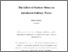# The Effect of Surface Stress on Interfacial Solitary Waves

Grundy, Dane (2019) The Effect of Surface Stress on Interfacial Solitary Waves. Doctoral thesis, University of East Anglia.Preview PDF Download (1MB) | Preview

## Abstract

A range of physical problems involving solitary wave solutions to the Korteweg-de Vries (KdV) equation are discussed. More specifically, relevance of perturbed forms of the Korteweg-de Vries equation and the effect of surface stress on the propagation of long-wavelength disturbances on the surface of a fluid layer of finite depth is considered. A weakly nonlinear analysis is performed leading to an evolution equation similar to the classic KdV equation, but modified by additional terms due to the viscosity and to the tangential and normal stress at the surface.

A thorough numerical analysis is constructed concerning numerical solutions to the KdV equation including an extensive stability analysis. Solutions which are perturbations of travelling solitary wave solutions are then considered which is mainly focussed on the Korteweg-de Vries-Burgers’ (KdV-B) equation. Asymptotic analyses demonstrate the appearance of a decaying oscillatory tail behind a core solitary wave-like solution. The solution in the tail region is determined in the form of a convolution integral involving the Airy function, while the core solution is obtainable explicitly.

Attention is also focussed on the effect of stress at the fluid surface. Constant surface tension leads to a normal stress at the surface, but the presence of an insoluble surfactant or the application of an electric field can also give rise to tangential stresses. In the large Reynolds number limit, the governing equation for the surface elevation contains contributions from two boundary layers in the flow: one is adjacent to the free surface while the other lies at the base of the fluid layer.

All asymptotic results are fully validated by comparison with numerical results obtained using a pseudospectral numerical integrating factor scheme.

Item Type: Thesis (Doctoral) Faculty of Science > School of Mathematics Jennifer Whitaker 20 Nov 2019 15:04 20 Nov 2019 15:04 https://ueaeprints.uea.ac.uk/id/eprint/73007View Item